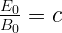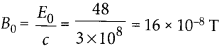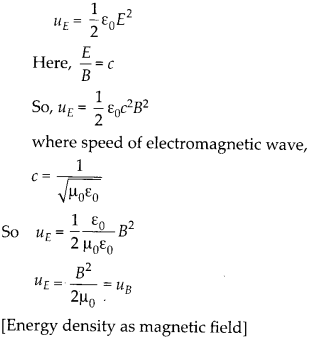Enlightened

# Question 10: NCERT Solutions for 12th Class Physics: Chapter 8-Electromagnetic Waves

• 0

Question 10: NCERT Solutions for 12th Class Physics: Chapter 8-Electromagnetic Waves

In a plane electromagnetic wave, the electric field oscillates sinusoidally at a frequency of 2.0 × 1010 Hz and amplitude 48 Vm-1.
(a) What is the wavelength of the wave?
(b) What is the amplitude of the oscillating magnetic field?
(c) Show that the average energy density of the E field equals the average energy density of the B field, [c = 3 × 108 m s-1.]

Share

1. Solution:
(a) Wavelength of electromagnetic wave is(b) Amplitude of magnetic field,(c) Energy density as electric field,Check the complete chapter with solutions.

NCERT Solutions for 12th Class Physics: Chapter 8-Electromagnetic Waves

• 0